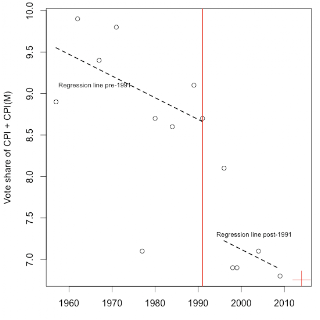# The Decline of the Left

riting in Business Standard today, Surjit Bhalla has a table of the vote share of the CPI and the CPI(M) put together. I thought it would be useful to see this data as a time-series, so here is the result.

Click on the graph to see it more clearly. Each circle is a data point. The dashed line is a (robust) regression with a shift in the intercept in 1991, reflecting the fall of communism. As we can see, the fall of communism seems to have gone along with a loss of vote share of 1.3 percentage points for the Left.

The latest result is a bit worse than the trend line might have suggested: tactical factors went a bit against the Left. At the same time, the CPI and CPI(M) leadership can take heart: the latest result is not all that far from the historic decline of the left, so this does not suggest that the leadership made particularly large tactical errors. What they are perhaps up against is historical forces.

The red coloured plus sign is the linear extrapolation for 2014; the slope implies losing roughly 0.13 percentage points of vote share each five years. (The statistical signifiance is weak; it’s a t stat of -1.52).Here’s the R code which you can experiment with:

```library(MASS) dates <- c(57,62,67,71,77,80,84,89,91,96,98,99,104,109)+1900 vshare <- c(8.9,9.9,9.4,9.8,7.1,8.7,8.6,9.1,8.7,8.1,6.9,6.9,7.1,6.8) post1991 <- dates > 1991 m <- rlm(vshare ~ -1 + dates + post1991) summary(m) m\$coefficients*5 # lose this much each gen. election```

png(”ic.png”, width=550,height=550, pointsize=16)
par(mai=c(.8,1.1,.2,.2))
plot(dates, vshare, type=”p”, xlim=c(1957,2014), xlab=”", ylab=”Vote share of CPI + CPI(M)”)
lines(dates[1:9], fitted.values(m)[1:9], lty=2, lwd=2)
lines(dates[10:14], fitted.values(m)[10:14], lty=2, lwd=2)
abline(v=1991, col=”red”, lwd=2)
points(2014, m\$coefficients %*% c(2014,0,1), cex=3, col=”red”, pch=3)
text(1966,9.1,”Regression line pre-1991″,cex=.7)
text(2003,7.3,”Regression line post-1991″, cex=.7)

### 2 comments to The Decline of the Left

• There’s about as much correlation there as a Jackson Pollock painting.

• SteveMDFP

To a typical reader, this is all opaque. It is not at all obvious that you’re referring to politics in India. It is not at all obvious that the definition of “Left” here is essentially the communist parties of India.

Does it make any sense at all to even calculate a trend line for votes for a political movement? Parties decline or advance based in large part on their particular leaders at any given time.